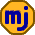## Sunday, August 23, 2009

### Understanding Investment Risk and Volatility

This is a Sunday feature looking back at selected articles from the early days of this blog before readership had ramped up. Enjoy.

In a previous post, I showed how the average real return in the U.S. stock market from 1926 to 2000 is 9.3%, but that this translated into a compounded real return of only 7.4%. The reason for this difference is the volatility of the returns. Let’s go for a better understanding of the cost of volatility without any advanced math.

A Simple Example

Suppose that you have \$10,000 invested for two years. In the first year you lose 10%, and the next year you make 10%. It might seem at first that you have your \$10,000 back, but that isn’t exactly right. After the first year you were down to \$9000, and then in the second year you earned 10% on that \$9000 to get a total of \$9900. In the end you lost 1% of your money.

However, the annual returns were -10% and +10% for an average return of 0%. The lost 1% over the two years is not due to a negative expected return; it is due to the volatility of the returns. The average return is 0%, but the compounded return is about -0.5% per year.

Another Example

Extreme examples are sometimes useful to bring out subtle points. Let’s consider an example that is unlikely to exist in the real world. Suppose you find an investment that returns either +100% or -60% with equal chance after a month. Each month either you win and your money doubles or you lose and 60% of your money disappears.

On the surface, this sounds great. Adding up the returns and dividing by 2, we see that the average return is 20% each month. If you could compound \$10,000 at 20% per month for 3 years, you would have \$7 million! You decide to go for it, and here is the result:What happened to the millions? The original \$10,000 is down to \$180. A first thought is that due to bad luck, the investment returned -60% more often than +100%. But, it turns out that both happened exactly 18 times.

To understand this, we need to think about it in a different way. Instead of +100%, think of it as multiplied by 2. Instead of -60%, think of it as leaving you with 40%, which is the same as divided by 2.5. So your investment is multiplied by 2 half the time and divided by 2.5 half the time.

Now it makes perfect sense that the investment value would drop. Starting with \$10,000, after one rise (to \$20,000), and then one drop (to \$8000), we have lost 20%. Over 3 years, this happened 18 times. The compounded annual return is -10.6%.

Does all this mean that the original calculation of the average return being +20% and expecting to have \$7 million at the end is wrong? No, it doesn’t. If you repeated this experiment enough times, you would find that on average you would really get \$7 million. In over 98% of the trials the return would be less than average, but in a very small fraction of the trials the return would be an outrageously large amount. It is these large amounts that inflate the average to \$7 million.

The most likely outcome is still that you will lose most of your money. In real life, this effect isn’t as dramatic, but the idea is the same. The average stock market return from 1926 to 2000 was 9.3%, but due to the volatility of the returns, the compounded return was only 7.4%.

1.Other stated, 1) the sequence of returns matters more and more the more volatile the retunrs. 2) reducing volatility is a very desirable portfolio goal.

2.Cabadian Investor: If by "sequence" you mean the order of the yearly returns, the sequence doesn't affect the final result (unless the investor loses his cool and bails out). However, the volatility, which is a measure of the degree to which each time period's returns deviate from their average, does matter quite a bit. Reducing volatility is definitely a desirable goal as long as you don't give up too much of your expected return.

3.Good distinction between average and compound returns. Your extreme example may not be too far off the possibilities for investors in triple-leveraged ETFs.

4.Larry: You're right about the extreme volatility of leveraged ETFs. I doubt that very many investors in these products understand the volatility penalty.